## 机器学习：划分聚类方法实现与应用

6981 字 · 47 阅读 · 2023 年 05 月 13 日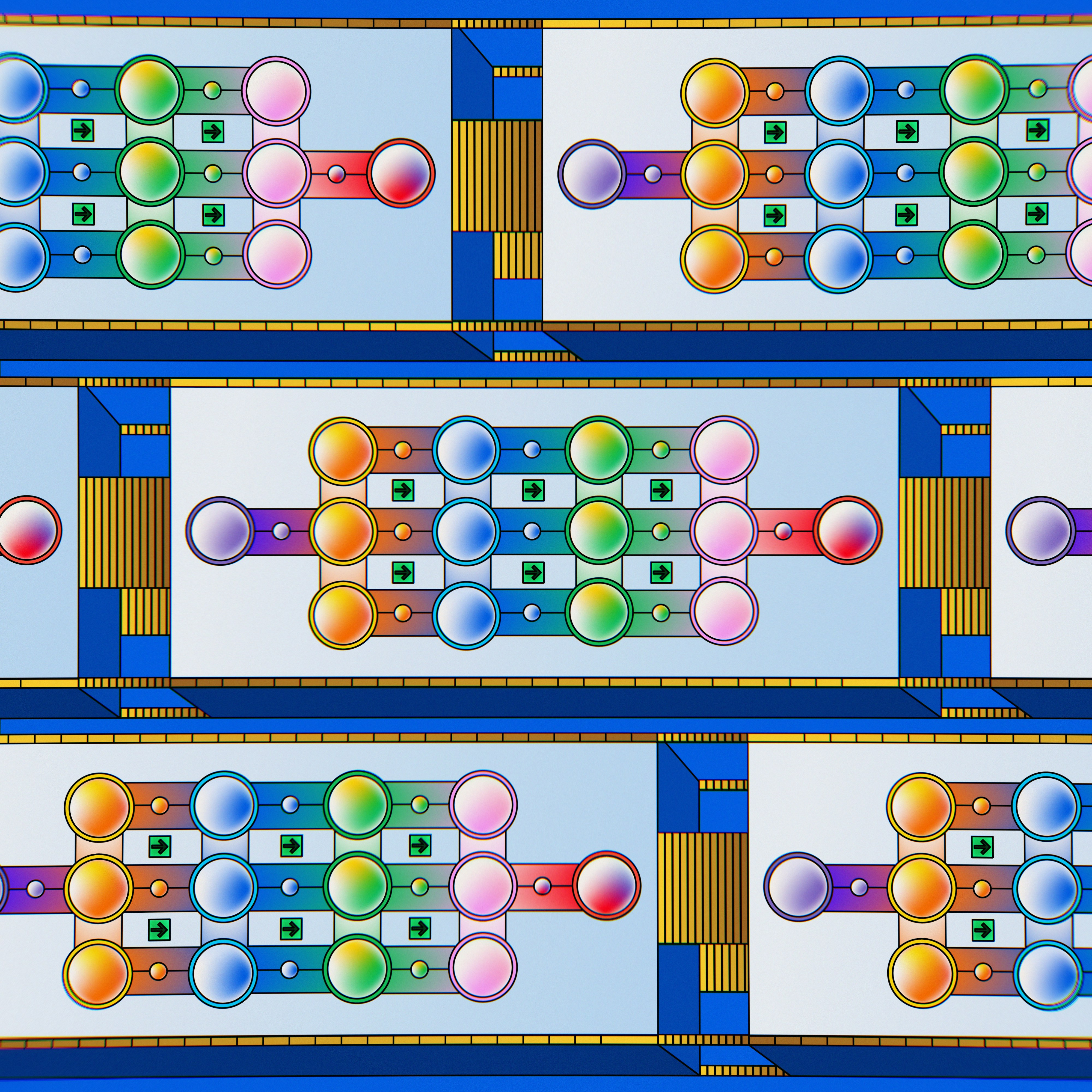### 知识点

• 划分聚类介绍
• K-Means 聚类方法
• 中心点移动过程可视化
• K-Means++ 算法实现

### K-Means 聚类方法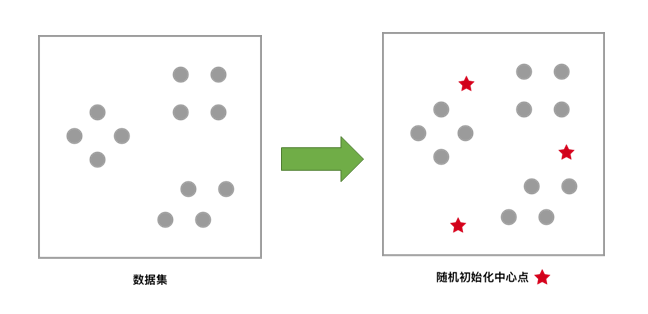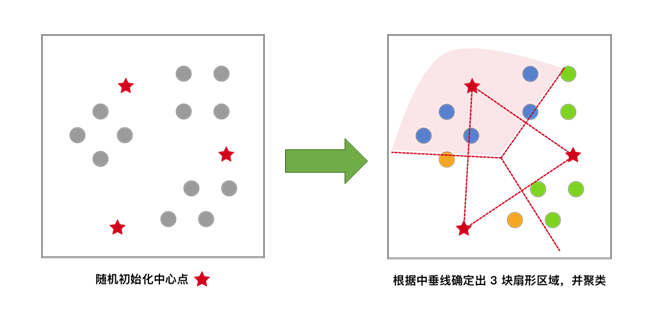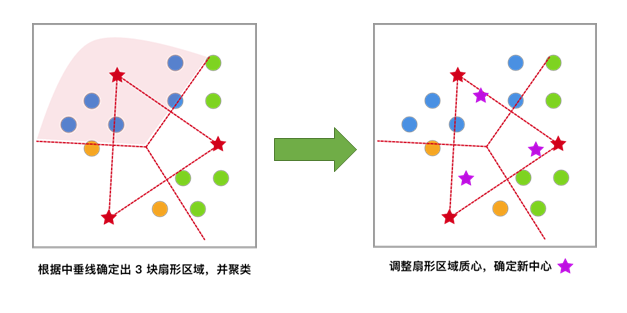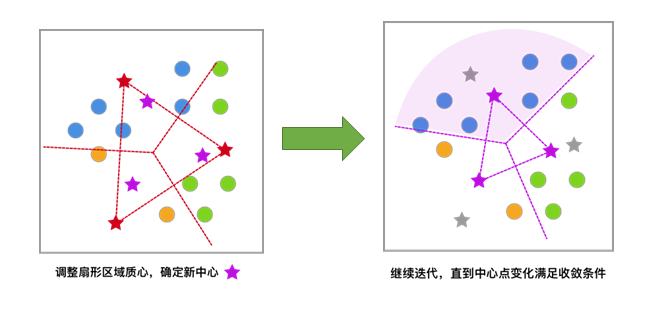### 生成示例数据

data,label = sklearn.datasets.make_blobs(n_samples=100,n_features=2,centers=3,center_box=(-10.0,10.0),random_state=None)


• n_samples：表示生成数据总个数,默认为 100 个。
• n_features：表示每一个样本的特征个数，默认为 2 个。
• centers：表示中心点的个数，默认为 3 个。
• center_box：表示每一个中心的边界,默认为 -10.0 到 10.0。
• random_state：表示生成数据的随机数种子。

• data：表示数据信息。
• label：表示数据类别。

from sklearn.datasets import make_blobs

# 构造示例数据
blobs, _ = make_blobs(n_samples=200, centers=3, random_state=18)
blobs[:10]  # 打印出前 10 条数据的信息


import matplotlib.pyplot as plt
%matplotlib inline

plt.scatter(blobs[:, 0], blobs[:, 1], s=20)  # 数据展示


### 随机初始化中心点

import numpy as np

def random_k(k, data):
"""
参数:
k -- 中心点个数
data -- 数据集

返回:
init_centers -- 初始化中心点
"""
# 初始化中心点
prng = np.random.RandomState(27)  # 定义随机种子
num_feature = np.shape(data)
# 初始化从标准正态分布返回的一组随机数，为了更加贴近数据集这里乘了一个 5
init_centers = prng.randn(k, num_feature)*5
return init_centers

init_centers = random_k(3, blobs)
init_centers


# 初始中心点展示
plt.scatter(blobs[:, 0], blobs[:, 1], s=20)
plt.scatter(init_centers[:, 0], init_centers[:, 1], s=100, marker='*', c="r")


### 计算样本与中心点的距离

def d_euc(x, y):
"""
参数:
x -- 数据 a
y -- 数据 b

返回:
d -- 数据 a 和 b 的欧氏距离
"""
# 计算欧氏距离
d = np.sqrt(np.sum(np.square(x - y)))
return d


### 最小化 SSE，更新聚类中心

$$SSE(C)=\sum_{k=1}^{K}\sum_{x_{i}\in C_{k}}\left \| x_{i}-c_{k} \right \|^{2} \tag{2}$$

$$c_{k}=\frac{\sum_{x_{i} \in C_{k}}x_{i}}{I(C_{k})} \tag{3}$$

$I(C_{k})$ 表示在第 $k$ 个集合 $C_{k}$ 中数据的个数。

def update_center(clusters, data, centers):
"""
参数:
clusters -- 每一点分好的类别
data -- 数据集
centers -- 中心点集合

返回:
new_centers.reshape(num_centers,num_features) -- 新中心点集合
"""
# 中心点的更新
num_centers = np.shape(centers)  # 中心点的个数
num_features = np.shape(centers)  # 每一个中心点的特征数
container = []
for x in range(num_centers):
each_container = []
container.append(each_container)  # 首先创建一个容器,将相同类别数据存放到一起

for i, cluster in enumerate(clusters):
container[cluster].append(data[i])

# 为方便计算，将 list 类型转换为 np.array 类型
container = np.array(list(map(lambda x: np.array(x), container)))

new_centers = np.array([])  # 创建一个容器，存放中心点的坐标
for i in range(len(container)):
each_center = np.mean(container[i], axis=0)  # 计算每一子集中数据均值作为中心点
new_centers = np.append(new_centers, each_center)

return new_centers.reshape(num_centers, num_features)  # 以矩阵的方式返回中心点坐标


### K-Means 聚类算法实现

K-Means 算法则采用的是迭代算法，避开优化 SSE 函数，通过不断移动中心点的距离，最终达到聚类的效果。首先来看一下算法流程：

1. 初始化中心点：判断数据集可能被分为 $k$ 个子集，随机生成 $k$ 个随机点作为每一个子集的中心点。
2. 距离计算，类别标记：样本和每一个中心点进行距离计算，将距离最近的中心点所代表的类别标记为该样本的类别。
3. 中心点位置更新：计算每一个类别中的所有样本的均值，作为新的中心点位置。
4. 重复 2，3 步骤，直到中心点位置不再变化。

def kmeans_cluster(data, init_centers, k):
"""
参数:
data -- 数据集
init_centers -- 初始化中心点集合
k -- 中心点个数

返回:
centers_container -- 每一次更新中心点的集合
cluster_container -- 每一次更新类别的集合
"""
# K-Means 聚类
max_step = 50  # 定义最大迭代次数，中心点最多移动的次数。
epsilon = 0.001  # 定义一个足够小的数，通过中心点变化的距离是否小于该数，判断中心点是否变化。

old_centers = init_centers

centers_container = []  # 建立一个中心点容器，存放每一次变化后的中心点，以便后面的绘图。
cluster_container = []  # 建立一个分类容器，存放每一次中心点变化后数据的类别
centers_container.append(old_centers)

for step in range(max_step):
cluster = np.array([], dtype=int)
for each_data in data:
distances = np.array([])
for each_center in old_centers:
temp_distance = d_euc(each_data, each_center)  # 计算样本和中心点的欧式距离
distances = np.append(distances, temp_distance)
lab = np.argmin(distances)  # 返回距离最近中心点的索引，即按照最近中心点分类
cluster = np.append(cluster, lab)
cluster_container.append(cluster)

new_centers = update_center(cluster, data, old_centers)  # 根据子集分类更新中心点

# 计算每个中心点更新前后之间的欧式距离
difference = []
for each_old_center, each_new_center in zip(old_centers, new_centers):
difference.append(d_euc(each_old_center, each_new_center))

if (np.array(difference) < epsilon).all():  # 判断每个中心点移动是否均小于 epsilon
return centers_container, cluster_container

centers_container.append(new_centers)
old_centers = new_centers

return centers_container, cluster_container


# 计算最终中心点
centers_container, cluster_container = kmeans_cluster(blobs, init_centers, 3)
final_center = centers_container[-1]
final_cluster = cluster_container[-1]
final_center


# 可视化展示
plt.scatter(blobs[:, 0], blobs[:, 1], s=20, c=final_cluster)
plt.scatter(final_center[:, 0], final_center[:, 1], s=100, marker='*', c="r")


num_axes = len(centers_container)

fig, axes = plt.subplots(1, num_axes, figsize=(20, 4))

axes.scatter(blobs[:, 0], blobs[:, 1], s=20, c=cluster_container)
axes.scatter(init_centers[:, 0], init_centers[:, 1],
s=100, marker='*', c="r")
axes.set_title("initial center")

for i in range(1, num_axes-1):
axes[i].scatter(blobs[:, 0], blobs[:, 1], s=20, c=cluster_container[i])
axes[i].scatter(centers_container[i][:, 0],
centers_container[i][:, 1], s=100, marker='*', c="r")
axes[i].set_title("step {}".format(i))

axes[-1].scatter(blobs[:, 0], blobs[:, 1], s=20, c=cluster_container[-1])
axes[-1].scatter(final_center[:, 0], final_center[:, 1],
s=100, marker='*', c="r")
axes[-1].set_title("final center")


• 初始化中心点的位置很好，比较均匀分布在了数据范围中。如果初始化中心点集中分布在某一角落，迭代次数肯定会增加。
• 示例数据分布规整和简单，使得无需迭代多次就能收敛。

### K-Means 算法聚类中的 K 值选择

sklearn.cluster.k_means(X, n_clusters)


• X：表示需要聚类的数据。
• n_clusters：表示聚类的个数，也就是 K 值。

• centroid：表示中心点坐标。
• label：表示聚类后每一个样本的类别。
• inertia：每一个样本与最近中心点距离的平方和，即 SSE。
# 用 scikit-learn 聚类并绘图
from sklearn.cluster import k_means
model = k_means(blobs, n_clusters=5)

centers = model
clusters_info = model
plt.scatter(blobs[:, 0], blobs[:, 1], s=20, c=clusters_info)
plt.scatter(centers[:, 0], centers[:, 1], s=100, marker='*', c="r")


index = []  # 横坐标数组
inertia = []  # 纵坐标数组

# K 从 1~ 6 聚类
for i in range(6):
model = k_means(blobs, n_clusters=i + 1)
index.append(i + 1)
inertia.append(model)

# 绘制折线图
plt.plot(index, inertia, "-o")


### K-Means++ 聚类算法

blobs_plus, _ = make_blobs(n_samples=800, centers=5, random_state=18)  # 生成数据
plt.scatter(blobs_plus[:, 0], blobs_plus[:, 1], s=20)  # 将数据可视化展示


### 随机初始化中心点

km_init_center = random_k(5, blobs_plus)

plt.scatter(blobs_plus[:, 0], blobs_plus[:, 1], s=20)
plt.scatter(km_init_center[:, 0],
km_init_center[:, 1], s=100, marker='*', c="r")


### K-Means 聚类

km_centers, km_clusters = kmeans_cluster(blobs_plus, km_init_center, 5)
km_final_center = km_centers[-1]
km_final_cluster = km_clusters[-1]
plt.scatter(blobs_plus[:, 0], blobs_plus[:, 1], s=20, c=km_final_cluster)
plt.scatter(km_final_center[:, 0],
km_final_center[:, 1], s=100, marker='*', c="r")


plt.scatter(blobs_plus[:, 0], blobs_plus[:, 1], s=20, c=_)


### K-Means++ 算法流程

K-Means++ 相较于 K-Means 在初始化中心点上做了改进，在其他方面和 K-Means 相同。

1. 在数据集中随机选择一个样本点作为第一个初始化的聚类中心。
2. 计算样本中的非中心点与最近中心点之间的距离 $D(x)$ 并保存于一个数组里，将数组中的这些距离加起来得到 $sum(D(x))$ 。
3. 取一个落在 $sum(D(x))$ 范围中的随机值 $R$ ，重复计算 $R=R-D(x)$ 直至得到 $R\leq0$ ，选取此时的点作为下一个中心点。
4. 重复 2,3 步骤，直到 $K$ 个聚类中心都被确定。
5. 对 $K$ 个初始化的聚类中心，利用 K-Means 算法计算最终的聚类中心。

### K-Means++ 算法实现

K-Means++ 在初始化样本点之后，计算其他样本与其最近的中心点距离之和，以备下一个中心点的选择，下面用 Python 来进行实现：

def get_sum_dis(centers, data):
"""
参数:
centers -- 中心点集合
data -- 数据集

返回:
np.sum(dis_container) -- 样本距离最近中心点的距离之和
dis_container -- 样本距离最近中心点的距离集合
"""

dis_container = np.array([])
for each_data in data:
distances = np.array([])
for each_center in centers:
temp_distance = d_euc(each_data, each_center)  # 计算样本和中心点的欧式距离
distances = np.append(distances, temp_distance)
lab = np.min(distances)
dis_container = np.append(dis_container, lab)
return np.sum(dis_container), dis_container


def get_init_center(data, k):
"""
参数:
data -- 数据集
k -- 中心点个数

返回:
np.array(center_container) -- 初始化中心点集合
"""
# K-Means++ 初始化中心点
seed = np.random.RandomState(20)
p = seed.randint(0, len(data))
first_center = data[p]

center_container = []
center_container.append(first_center)

for i in range(k-1):
sum_dis, dis_con = get_sum_dis(center_container, data)
r = np.random.randint(0, sum_dis)
for j in range(len(dis_con)):
r = r - dis_con[j]
if r <= 0:
center_container.append(data[j])
break
else:
pass

return np.array(center_container)


plus_init_center = get_init_center(blobs_plus, 5)
plus_init_center


num = len(plus_init_center)

fig, axes = plt.subplots(1, num, figsize=(25, 4))

axes.scatter(blobs_plus[:, 0], blobs_plus[:, 1], s=20, c="b")
axes.scatter(plus_init_center[0, 0], plus_init_center[0, 1],
s=100, marker='*', c="r")
axes.set_title("first center")

for i in range(1, num):
axes[i].scatter(blobs_plus[:, 0], blobs_plus[:, 1], s=20, c="b")
axes[i].scatter(plus_init_center[:i+1, 0],
plus_init_center[:i+1, 1], s=100, marker='*', c="r")
axes[i].set_title("step{}".format(i))

axes[-1].scatter(blobs_plus[:, 0], blobs_plus[:, 1], s=20, c="b")
axes[-1].scatter(plus_init_center[:, 0],
plus_init_center[:, 1], s=100, marker='*', c="r")
axes[-1].set_title("final center")


plus_centers, plus_clusters = kmeans_cluster(blobs_plus, plus_init_center, 5)
plus_final_center = plus_centers[-1]
plus_final_cluster = plus_clusters[-1]

plt.scatter(blobs_plus[:, 0], blobs_plus[:, 1], s=20, c=plus_final_cluster)
plt.scatter(plus_final_center[:, 0],
plus_final_center[:, 1], s=100, marker='*', c="r")


### Mini-Batch K-Means 聚类算法

import time
from sklearn.cluster import MiniBatchKMeans, KMeans

test_data, _ = make_blobs(20000, n_features=2, cluster_std=0.9, centers=5, random_state=20)

km = KMeans(n_clusters=5)
mini_km = MiniBatchKMeans(n_clusters=5)

fig, axes = plt.subplots(nrows=1, ncols=2, figsize=(10, 5))

for i, model in enumerate([km, mini_km]):
t0 = time.time()
model.fit(test_data)
t1 = time.time()
t = t1 - t0
sse = model.inertia_
axes[i].scatter(test_data[:, 0], test_data[:, 1], c=model.labels_)
axes[i].set_xlabel("time: {:.4f} s".format(t))
axes[i].set_ylabel("SSE: {:.4f}".format(sse))

axes.set_title("K-Means")
axes.set_title("Mini Batch K-Means")


### 系列文章•